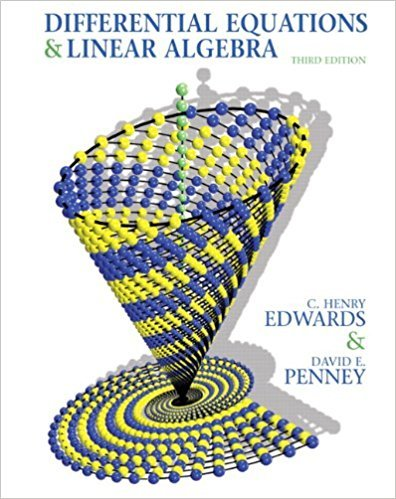×
×

# Solutions for Chapter 3.6: Differential Equations and Linear Algebra 3rd Edition## Full solutions for Differential Equations and Linear Algebra | 3rd Edition

ISBN: 9780136054252Solutions for Chapter 3.6

Solutions for Chapter 3.6
4 5 0 308 Reviews
18
5
##### ISBN: 9780136054252

Differential Equations and Linear Algebra was written by and is associated to the ISBN: 9780136054252. Chapter 3.6 includes 58 full step-by-step solutions. This expansive textbook survival guide covers the following chapters and their solutions. Since 58 problems in chapter 3.6 have been answered, more than 12750 students have viewed full step-by-step solutions from this chapter. This textbook survival guide was created for the textbook: Differential Equations and Linear Algebra, edition: 3.

Key Math Terms and definitions covered in this textbook
• Affine transformation

Tv = Av + Vo = linear transformation plus shift.

• Augmented matrix [A b].

Ax = b is solvable when b is in the column space of A; then [A b] has the same rank as A. Elimination on [A b] keeps equations correct.

• Basis for V.

Independent vectors VI, ... , v d whose linear combinations give each vector in V as v = CIVI + ... + CdVd. V has many bases, each basis gives unique c's. A vector space has many bases!

• Cholesky factorization

A = CTC = (L.J]))(L.J]))T for positive definite A.

• Cross product u xv in R3:

Vector perpendicular to u and v, length Ilullllvlll sin el = area of parallelogram, u x v = "determinant" of [i j k; UI U2 U3; VI V2 V3].

• Dot product = Inner product x T y = XI Y 1 + ... + Xn Yn.

Complex dot product is x T Y . Perpendicular vectors have x T y = O. (AB)ij = (row i of A)T(column j of B).

• Exponential eAt = I + At + (At)2 12! + ...

has derivative AeAt; eAt u(O) solves u' = Au.

• Four Fundamental Subspaces C (A), N (A), C (AT), N (AT).

Use AT for complex A.

• Hessenberg matrix H.

Triangular matrix with one extra nonzero adjacent diagonal.

• Inverse matrix A-I.

Square matrix with A-I A = I and AA-l = I. No inverse if det A = 0 and rank(A) < n and Ax = 0 for a nonzero vector x. The inverses of AB and AT are B-1 A-I and (A-I)T. Cofactor formula (A-l)ij = Cji! detA.

• Kronecker product (tensor product) A ® B.

Blocks aij B, eigenvalues Ap(A)Aq(B).

• Markov matrix M.

All mij > 0 and each column sum is 1. Largest eigenvalue A = 1. If mij > 0, the columns of Mk approach the steady state eigenvector M s = s > O.

• Multiplication Ax

= Xl (column 1) + ... + xn(column n) = combination of columns.

• Norm

IIA II. The ".e 2 norm" of A is the maximum ratio II Ax II/l1x II = O"max· Then II Ax II < IIAllllxll and IIABII < IIAIIIIBII and IIA + BII < IIAII + IIBII. Frobenius norm IIAII} = L La~. The.e 1 and.e oo norms are largest column and row sums of laij I.

• Outer product uv T

= column times row = rank one matrix.

• Plane (or hyperplane) in Rn.

Vectors x with aT x = O. Plane is perpendicular to a =1= O.

• Spanning set.

Combinations of VI, ... ,Vm fill the space. The columns of A span C (A)!

• Standard basis for Rn.

Columns of n by n identity matrix (written i ,j ,k in R3).

• Symmetric matrix A.

The transpose is AT = A, and aU = a ji. A-I is also symmetric.

• Vector space V.

Set of vectors such that all combinations cv + d w remain within V. Eight required rules are given in Section 3.1 for scalars c, d and vectors v, w.

×